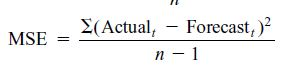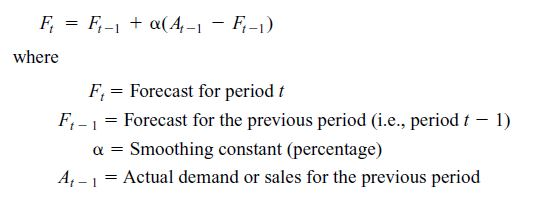# Solved: Problem 15-05 (Algorithmic) Consider the following time series data Week1 2 3 4 5 6

Problem 15-05 (Algorithmic) Consider the following time series data Week1 2 3 4 5 6 Value 16 12 14 13 15 14 a. Choose the correct time series plot. 20r Time Series Value 18 16 14 12 10 20r Time Series Value 18 16 12 10 Week(t) Week(t)

a) Ans (i)

The graph correctly depicts the value against the week entries. There is zig-zag pattern

Don't use plagiarized sources. Get Your Custom Essay on
Solved: Problem 15-05 (Algorithmic) Consider the following time series data Week1 2 3 4 5 6
GET AN ESSAY WRITTEN FOR YOU FROM AS LOW AS \$13/PAGE

b) The 3 week moving average is: (M1+M2+M3)/3

Example: Week 4 = (16+12+14)/3 = 14Week Value (D) Forecast (F) Error (D-F) Error Abs Error Sq 1 16 2 12 3 14 4 13 14.00 (1.00) 1.00 1 5 15 13.00 2.00 2.00 4 6 14 14.00 – – – 14.00

Week 7 forecast = 14

MSE = (1+4+0)/((3-1) = 2.5

c)Week Value (D) Forecast (F) Error (D-F) Error Abs Error Sq 1 16 2 12 16.00 3 14 15.20 4 13 14.96 (1.96) 1.96 3.84 5 15 14.57 0.43 0.43 0.19 6 14 14.65 (0.65) 0.65 0.43 14.52

Week 7 forecast = 14.52

MSE = (3.84+0.19+0.43)/(3-1) = 2.23

d) Exponential smoothing forecast is better due to lower MSE. Hence, less error